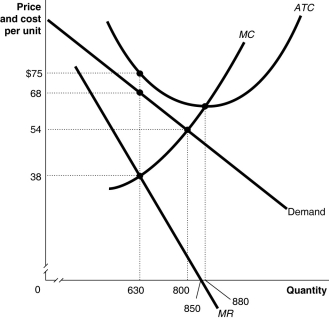# [Solved] Figure 15-3 Figure 15-3 Above Shows the Demand and Cost

Question 108
Multiple Choice

## Figure 15-3Figure 15-3 above shows the demand and cost curves facing a monopolist.-Refer to Figure 15-3. Suppose the monopolist represented in the diagram above produces positive output. What is the profit-maximizing/loss-minimizing output level?

A) 630 units
B) 800 units
C) 850 units
D) 880 units

10+ million students use Quizplus to study and prepare for their homework, quizzes and exams through 20m+ questions in 300k quizzes.

Explore our library and get Economics Homework Help with various study sets and a huge amount of quizzes and questions

3.5K

Study sets

66.5K

Quizzes

5.9M

Questions

Upload material to get free accessInvite a friend and get free accessSubscribe and get an instant access